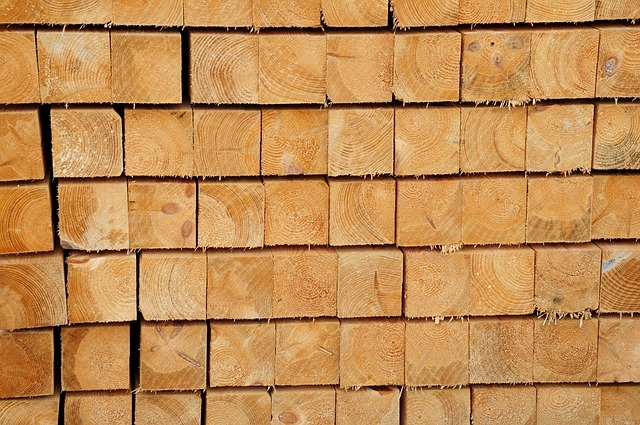# Manipulating Data Points# Introduction to Making Box Plots With Line Plots

This line plot and box plot represent the same data:

{0, 7, 13, 20}

1. Why doesn't the size of the box on the box plot line up with the data underneath it on the line plot?
2. What would happen to the "whiskers" if you changed the value of the lower or upper extreme values?
3. How are the box plot and line plot related?1 of 7